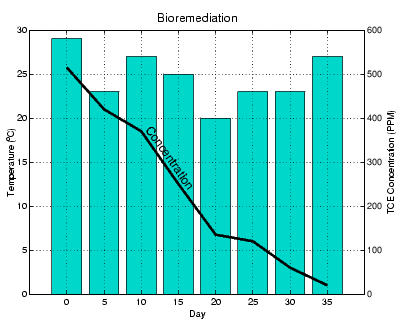GraphicsOverlaying Plots on Bar Graphs

You can overlay data on a bar graph by creating another axes in the same position. This enables you to have an independent y-axis for the overlaid dataset (in contrast to the `hold`` on` statement, which uses the same axes).

For example, consider a bioremediation experiment that breaks down hazardous waste components into nontoxic materials. The trichloroethylene (TCE) concentration and temperature data from this experiment are

• ```TCE = [515 420 370 250 135 120 60 20];
temp = [29 23 27 25 20 23 23 27];
```

This data was obtained from samples taken every five days during a thirty-five day period.

• ```days = 0:5:35;
```

Display a bar graph and label the x- and y-axis using the statements

• ```bar(days,temp)
xlabel('Day')
ylabel('Temperature (^{o}C)')
```

Overlaying a Line Plot on the Bar Graph

To overlay the concentration data on the bar graph, position a second axes at the same location as the first axes, but first save the handle of the first axes.

• ```h1 = gca;
```

Create the second axes at the same location before plotting the second dataset.

• ```h2 = axes('Position',get(h1,'Position'));
plot(days,TCE,'LineWidth',3)
```

To ensure that the second axes does not interfere with the first, locate the y-axis on the right side of the axes, make the background transparent, and set the second axes' x-tick marks to the empty matrix.

• ```set(h2,'YAxisLocation','right','Color','none','XTickLabel',[])
```

Align the x-axis of both axes and display the grid lines on top of the bars.

• ```set(h2,'XLim',get(h1,'XLim'),'Layer','top')```

Annotating the Graph.   These statements annotate the graph.

• ```text(11,380,'Concentration','Rotation'`,`-`55`,'FontSize',16)
ylabel('TCE Concentration (PPM)')
title('Bioremediation','FontSize',16)
```

To print the graph, set the current figure's `PaperPositionMode` to `auto`, which ensures the printed output matches the display.

• ``````set(gcf,'PaperPositionMode','auto')
``````Specifying X-Axis Data Area Graphs© 1994-2005 The MathWorks, Inc.